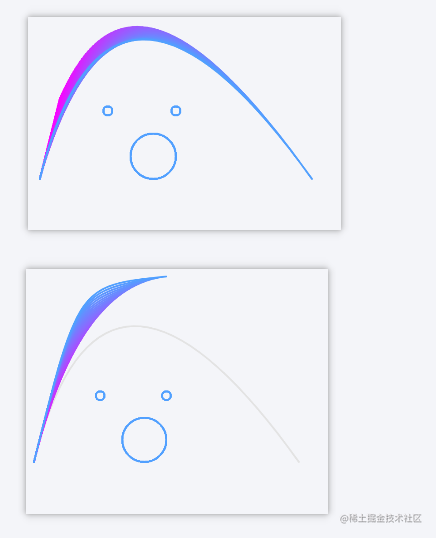# 【🎨万物皆可动】详解Canvas路径动画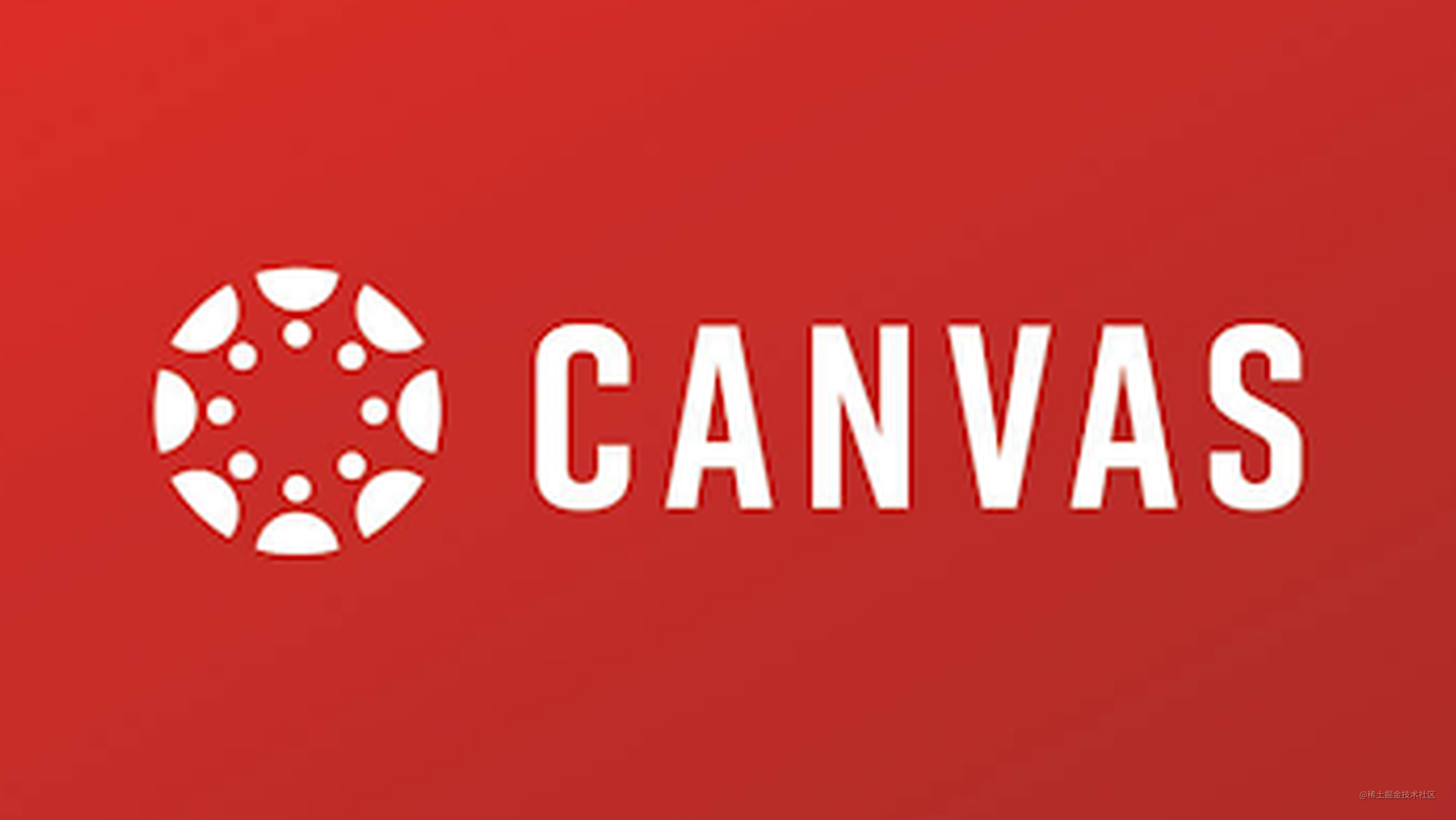## How——如何控制动画帧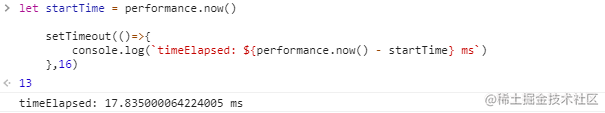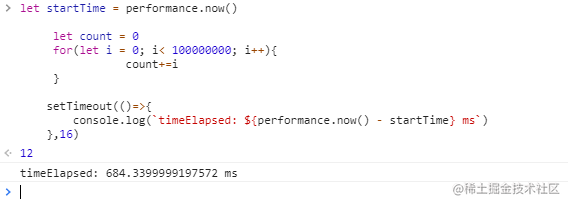requestAnimationFrame提供了更加平缓并且更加有效率的方式来执行动画，当系统准备好了重绘条件时才会调用我们动画帧的绘制方法。

requestAnimationFrame的使用可能听上去有点绕，但是不要紧，看完后面的这个例子应该就理解了。

## 从一根直线开始

``````<body>
<div id="executeButton" onclick="handleExecute()">执行</div>
<canvas id="myCanvas" width="800" height="800"></canvas>
<script>
function handleExecute() {
// 获取canvas元素
const canvas = document.querySelector('#myCanvas')
// 获取canvas渲染上下文
const ctx = canvas.getContext('2d')

// 设置线条样式
ctx.strokeStyle = 'rgba(81, 160, 255,1)'
ctx.lineWidth = 3
// 创建路径
ctx.beginPath()
// 移动笔触到(100,100)坐标处
ctx.moveTo(100,100)
// 把线连接到(700,700)这个位置
ctx.lineTo(700,700)
// 把刚刚的路径绘制出来
ctx.stroke()
}
</script>
</body>
``````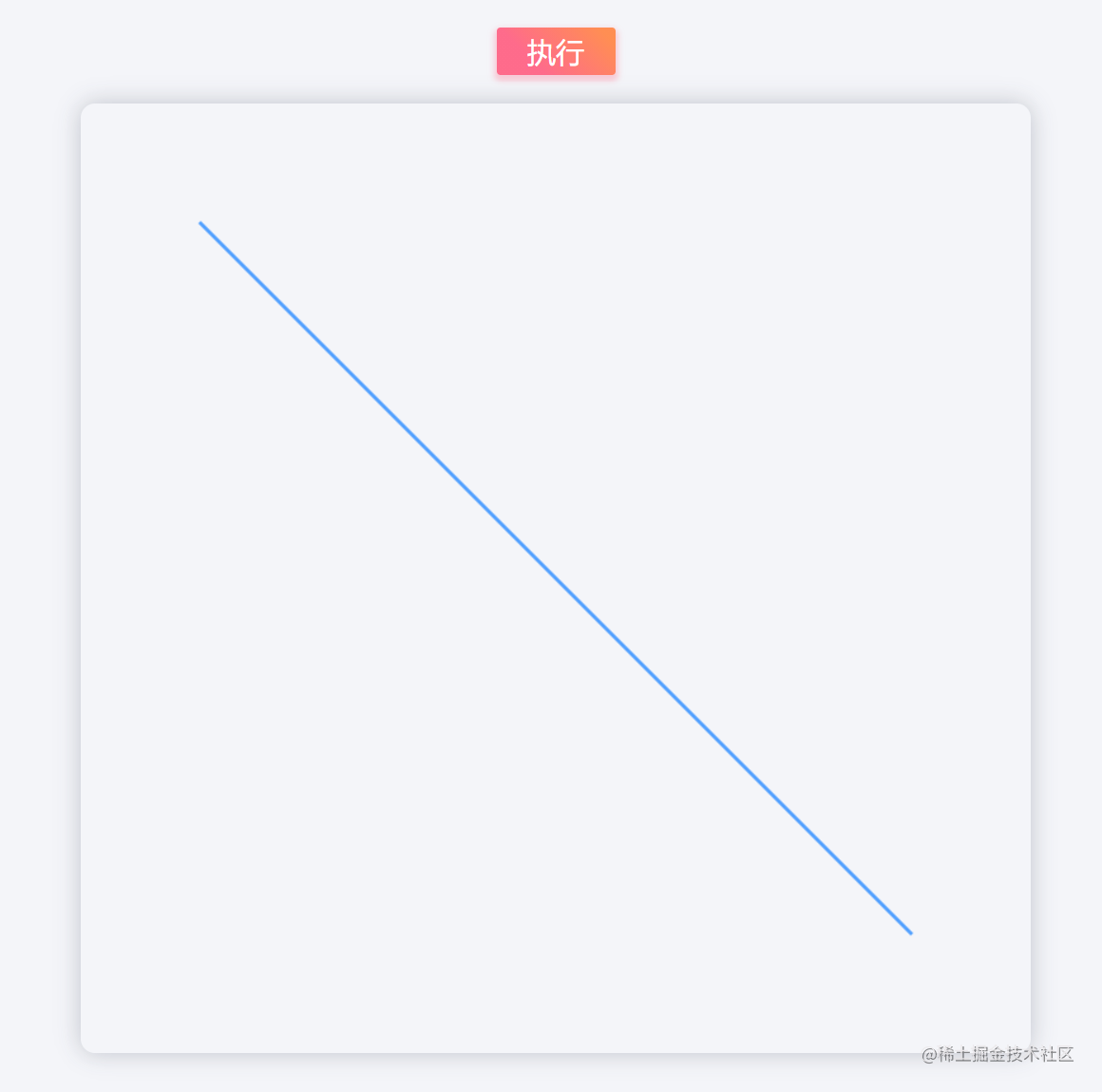codepen传送门codepen.io/channinghan…

1. 使用用多条路径，把这根线想象成若干个线段组成，每一次有序地绘制其中的一段，每一次的绘制就是一个动画帧。
2. 使用同一条路径，每一个动画帧绘制的直线长度逐渐增加，直到达到我们期望的长度。

#### 思路一：使用多条路径

progress为1时则表示已完成。当progress小于1时继续调用requestAnimationFrame来向浏览器请求我们绘制动画帧方法的执行。

``````<script>
function handleExecute() {
// 获取canvas元素
const canvas = document.querySelector('#myCanvas')
// 获取canvas渲染上下文
const ctx = canvas.getContext('2d')

// 设置线条样式
ctx.strokeStyle = 'rgba(81, 160, 255,1)'
ctx.lineWidth = 4
ctx.lineJoin = 'round'

// 定义起点和终点的坐标
const startX = 100
const startY = 100
const endX = 700
const endY = 700
let prevX = startX
let prevY = startY
let nextX
let nextY
// 第一帧执行的时间
let startTime;
// 期望动画持续的时间
const duration = 1000

/*
* 动画帧绘制方法.
* currentTime是requestAnimation执行回调方法step时会传入的一个执行时的时间(由performance.now()获得).
* */
const step = (currentTime) => {
// 第一帧绘制时记录下开始的时间
!startTime && (startTime = currentTime)
// 已经过去的时间(ms)
const timeElapsed = currentTime - startTime
// 动画执行的进度 {0,1}
const progress = Math.min(timeElapsed / duration, 1)

// 绘制方法
const draw = () => {
// 创建新的路径
ctx.beginPath()
// 创建子路径,并将起点移动到上一帧绘制到达的坐标点
ctx.moveTo(prevX, prevY)
// 计算这一帧中线段应该到达的坐标点,并且将prevX/Y更新为此值给下一帧使用.
prevX = nextX = startX + (endX - startX) * progress
prevY = nextY = startY + (endY - startY) * progress
// 用直线将刚刚moveTo中的点连接到(nextX,nextY)上
ctx.lineTo(nextX, nextY)
ctx.strokeStyle = `rgba(\${81}, \${160}, \${255},\${0.25})`
// 把这一帧的路径绘制出来
ctx.stroke()
}
draw()

if (progress < 1) {
requestAnimationFrame(step)
} else {
console.log('动画执行完毕')
}
}

requestAnimationFrame(step)
}
</script>
``````

codePen传送门codepen.io/channinghan…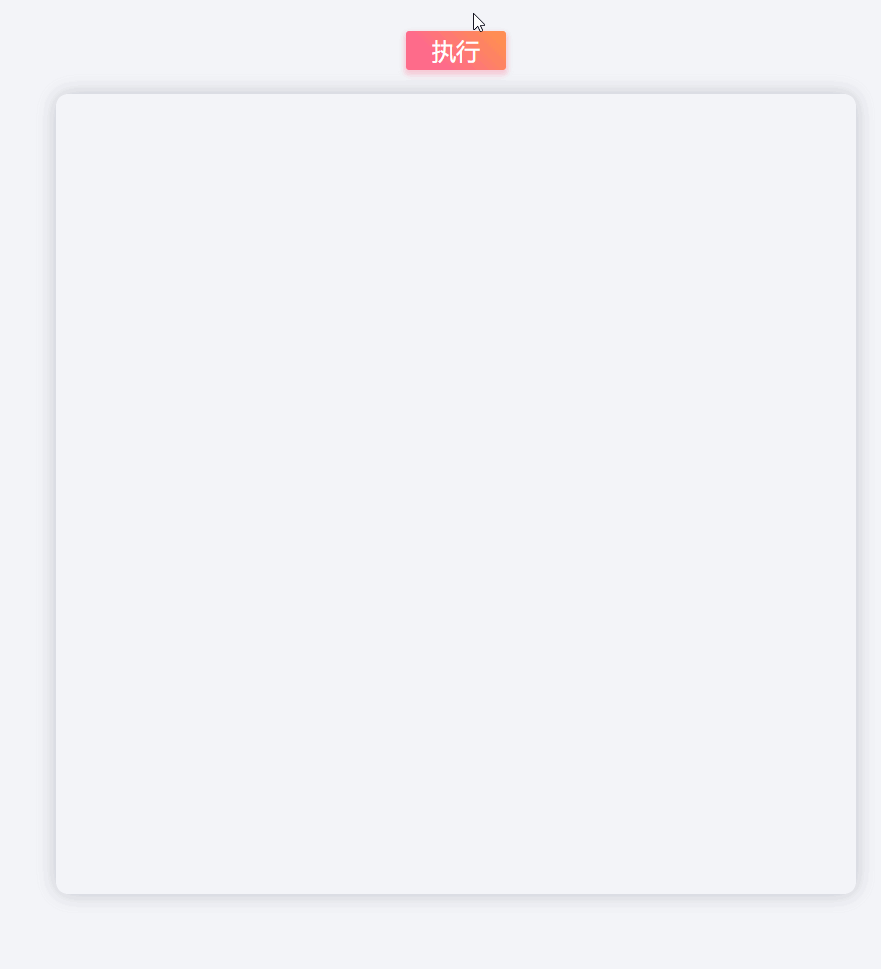#### 第二种：使用同一条路径

``````<script>
function handleExecute() {
// 获取canvas元素
const canvas = document.querySelector('#myCanvas')
// 获取canvas渲染上下文
const ctx = canvas.getContext('2d')

// 定义起点和终点的坐标
const startX = 100
const startY = 100
const endX = 700
const endY = 700
let nextX
let nextY

// 第一帧执行的时间
let startTime;
// 期望动画持续的时间
const duration = 1000

// 创建路径
ctx.beginPath()
// 创建一条子路径,把新的子路径起始点移动到(prevX,prevY)坐标.
ctx.moveTo(startX, startY)
// 设置线条样式
ctx.strokeStyle = `rgba(\${81}, \${160}, \${255},\${0.25})`
ctx.lineWidth = 4

/*
* 动画帧绘制方法.
* currentTime是requestAnimation执行回调方法step时会传入的一个执行时的时间(由performance.now()获得).
* */
const step = (currentTime) => {
// ctx.clearRect(startX - 4, startY - 4, Math.abs(endX - startY) + 8, Math.abs(endY - startY) + 8)
// 第一帧绘制时记录下开始的时间
!startTime && (startTime = currentTime)
// 已经过去的时间(ms)
const timeElapsed = currentTime - startTime
// 动画执行的进度 {0,1}
const progress = Math.min(timeElapsed / duration, 1)

// 绘制方法
const draw = () => {
// 计算这一帧中线段应该到达的坐标点
nextX = startX + (endX - startX) * progress
nextY = startY + (endY - startY) * progress
// 用直线连接子路径的最后的点到(nextX,nextY)坐标
ctx.lineTo(nextX, nextY)
// 绘制路径(所有子路径都会被绘制一次)
ctx.stroke()
}
draw()

if (progress < 1) {
requestAnimationFrame(step)
} else {
console.log('动画执行完毕')
}
}

requestAnimationFrame(step)
}
</script>
``````

codepen传送门codepen.io/channinghan…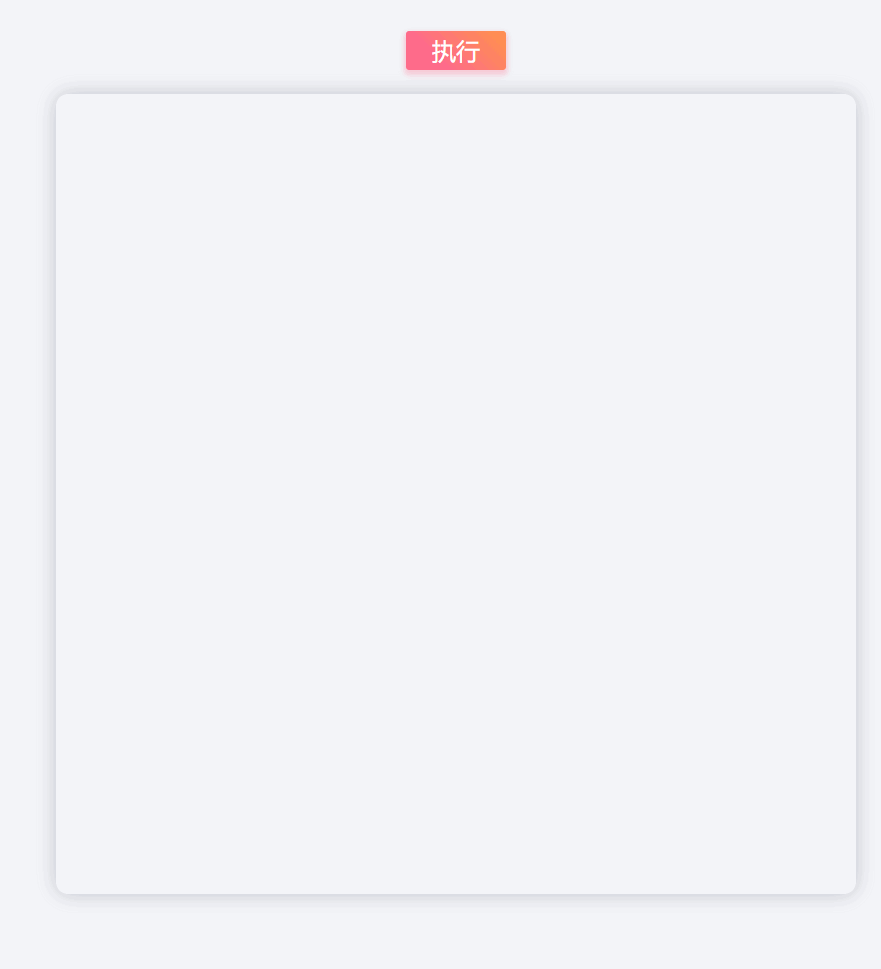### 加入缓动函数（easing function）

``````transition:  all 600ms ease-in-out;
``````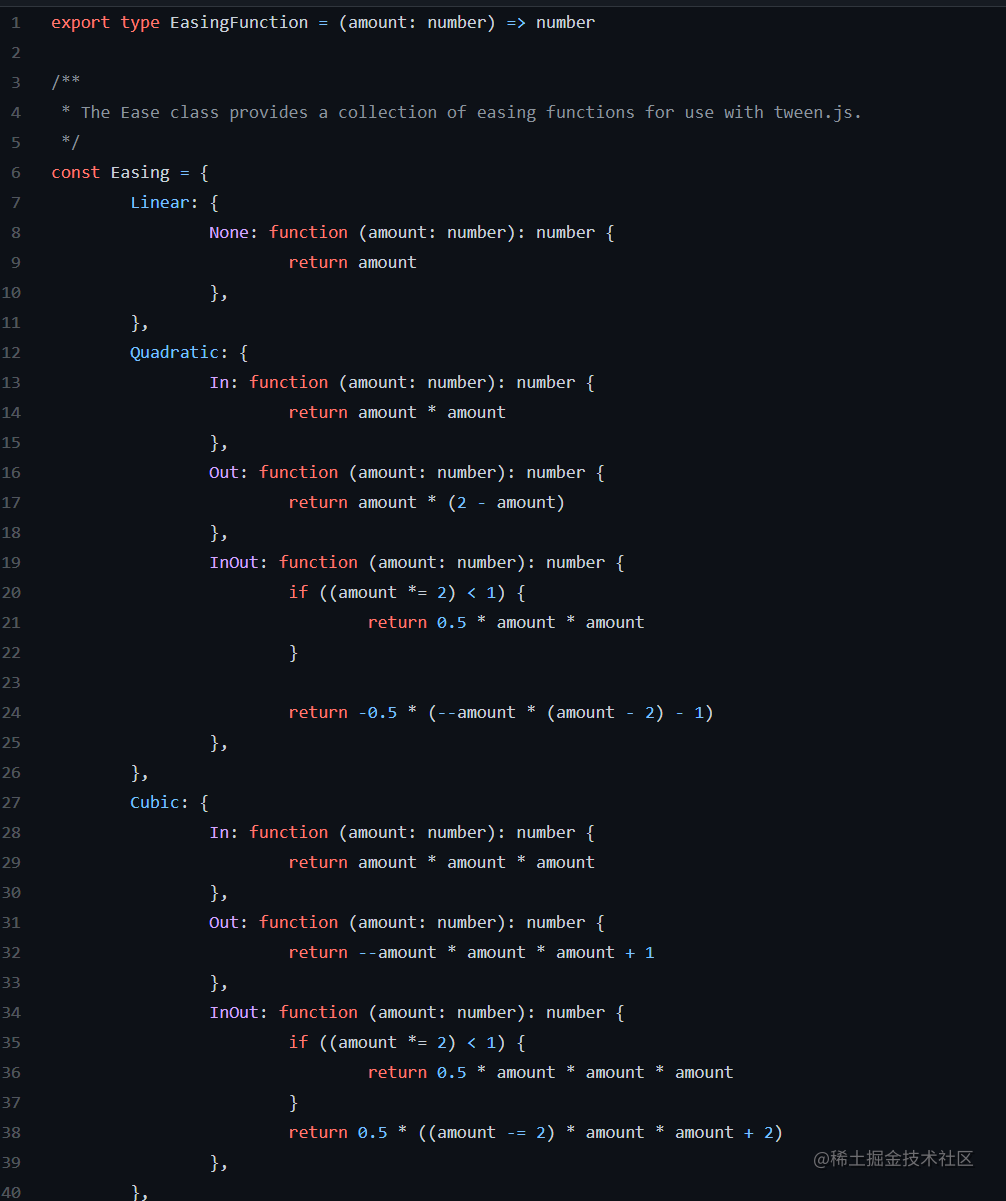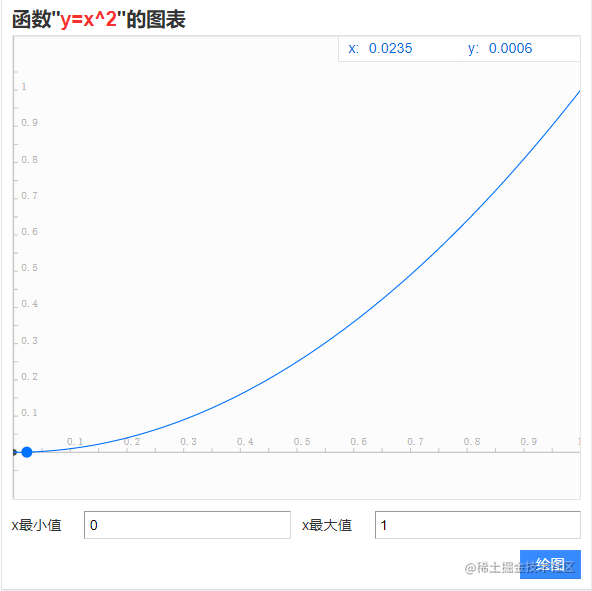``````let progress = Math.min(timeElapsed / duration, 1)
``````

codepen传送门codepen.io/channinghan…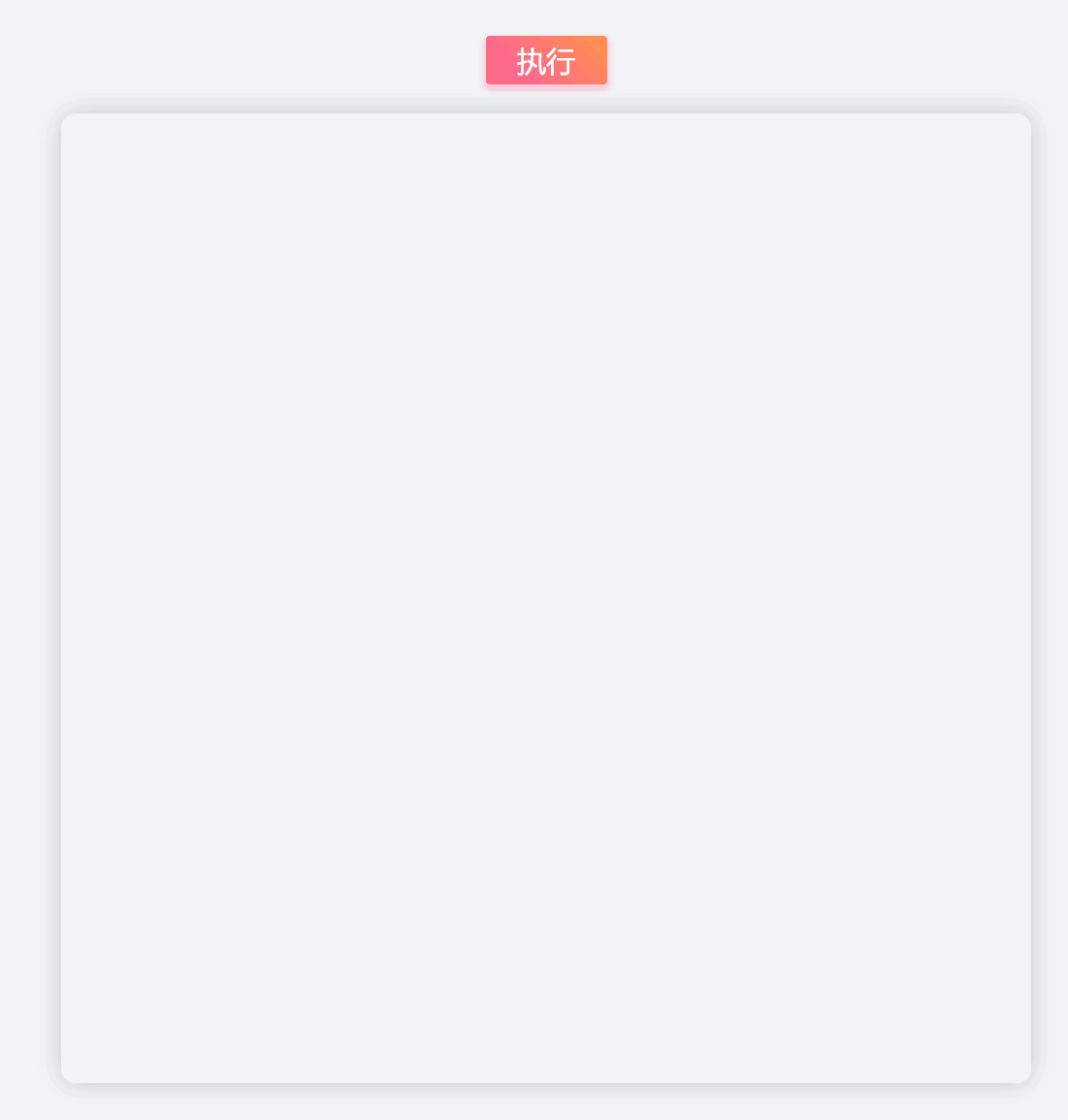### 在虚线中观察帧动画

``````<script>
function handleExecute() {
// ......

// 期望动画持续的时间
const duration = 1000
let count = 0

const step = (currentTime) => {
!startTime && (startTime = currentTime)
const timeElapsed = currentTime - startTime
const progress = Math.min(timeElapsed / duration, 1)

// 绘制方法
const draw = () => {
ctx.beginPath()
ctx.moveTo(prevX, prevY)

// 计算该次线段绘制的终点,并将prevX/Y更新为此值,给下一次绘制的时候使用
prevX = nextX = startX + (endX - startX) * progress
prevY = nextY = startY + (endY - startY) * progress

if (count % 2 === 0) {
// 设置线条样式
ctx.lineWidth = 20 * progress
ctx.strokeStyle = `rgba(\${171 * (1 - progress) + 81}, \${160 * progress}, \${255},1)`
ctx.lineTo(nextX, nextY)
ctx.stroke()
}
}
draw()

if (progress < 1) {
count++
requestAnimationFrame(step)
} else {
console.log('动画执行完毕')
console.log(`\${duration}ms内回调执行次数:\${count}次`)
}
}

// 向浏览器发送动画执行请求,当浏览器要进行重绘时,会调用我们传入的step方法
requestAnimationFrame(step)
}
</script>
``````

codepen传送门codepen.io/channinghan…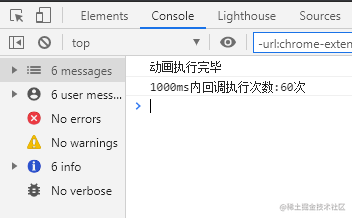## 折线

codepen传送门codepen.io/channinghan…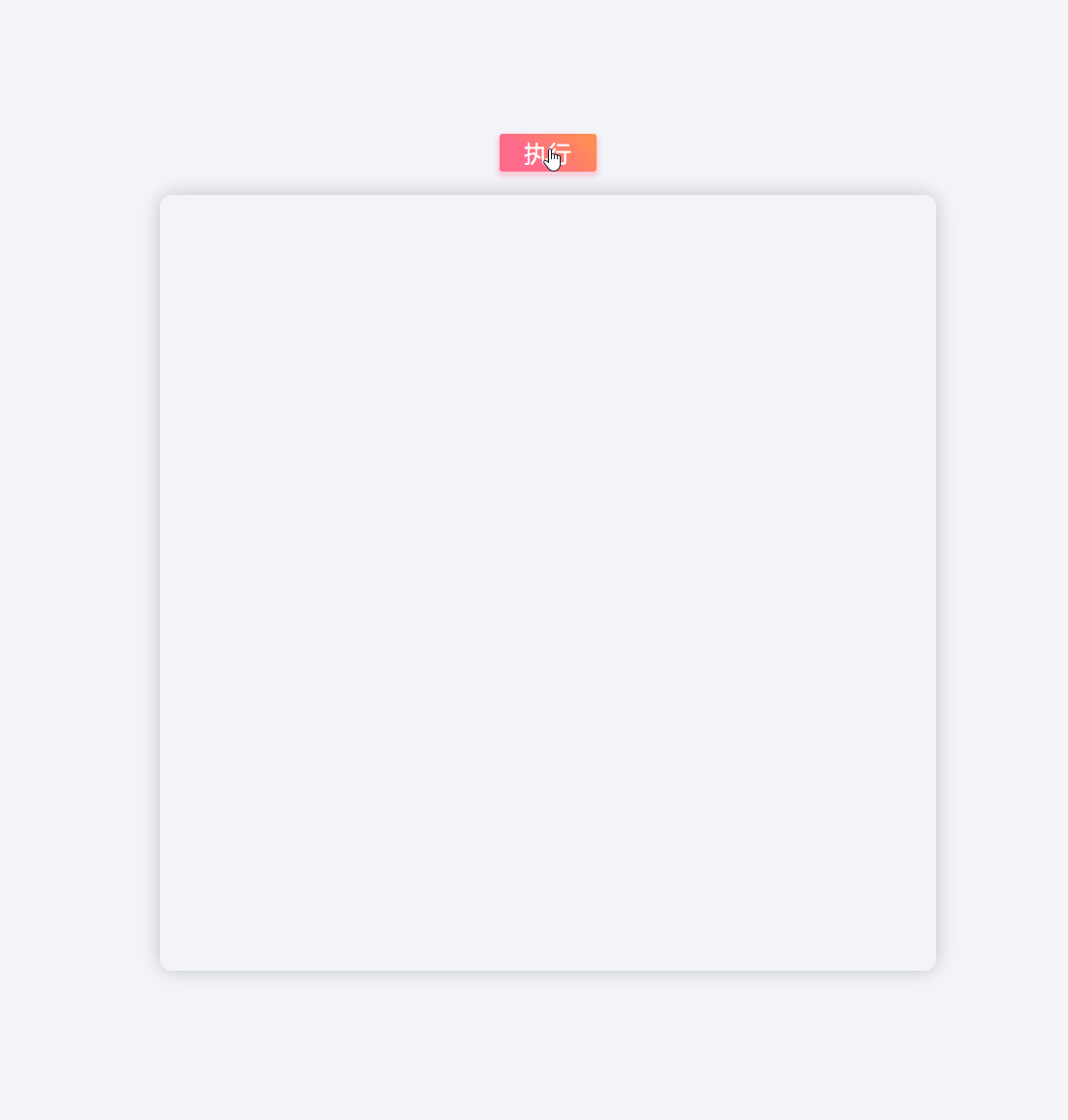`````` function handleExecute() {
const canvas = document.querySelector('#myCanvas')
const ctx = canvas.getContext('2d')

// 设置线条样式
ctx.lineWidth = 7
ctx.lineJoin = 'round'
ctx.lineCap = 'round'

// 顺序定义折线上各个转折点的坐标
const keyPoints = [
{x: 250, y: 200},
{x: 550, y: 200},
{x: 250, y: 500},
{x: 550, y: 500},
{x: 250, y: 200}
]
let prevX = keyPoints.x
let prevY = keyPoints.y
let nextX
let nextY
// 第一帧执行的时间
let startTime;
// 期望动画持续的时间
const duration = 900

// 动画被切分成若干段,每一段所占总进度的比例
const partProportion = 1 / (keyPoints.length - 1)
// 缓存绘制第n段线段的n值,为了在进行下一段绘制前把这一段线段的末尾补齐
let lineIndexCache = 1

/*
* 动画帧绘制方法.
* currentTime是requestAnimation执行回调方法step时会传入的一个执行时的时间(由performance.now()获得).
* */
const step = (currentTime) => {
// 第一帧绘制时记录下开始的时间
!startTime && (startTime = currentTime)
// 已经过去的时间(ms)
const timeElapsed = currentTime - startTime
// 动画执行的进度 {0,1}
let progress = Math.min(timeElapsed / duration, 1)
// 加入二次方缓动函数

// 描述当前所绘制的是第几段线段
const lineIndex = Math.min(Math.floor(progress / partProportion) + 1, keyPoints.length - 1)

//  当前线段的进度 {0,1}
const partProgress = (progress - (lineIndex - 1) * partProportion) / partProportion

// 绘制方法
const draw = () => {
ctx.strokeStyle = `rgba(\${81 + 175 * Math.abs(1 - progress * 2)}, \${160 - 160 * Math.abs(progress * 2 - 1)}, \${255},\${1})`
ctx.beginPath()
ctx.moveTo(prevX, prevY)
// 当绘制下一段线段前,把上一段末尾缺失的部分补齐
if (lineIndex !== lineIndexCache) {
ctx.lineTo(keyPoints[lineIndex - 1].x, keyPoints[lineIndex - 1].y)
lineIndexCache = lineIndex
}
prevX = nextX = ~~(keyPoints[lineIndex - 1].x + ((keyPoints[lineIndex]).x - keyPoints[lineIndex - 1].x) * partProgress)
prevY = nextY = ~~(keyPoints[lineIndex - 1].y + ((keyPoints[lineIndex]).y - keyPoints[lineIndex - 1].y) * partProgress)
ctx.lineTo(nextX, nextY)
ctx.stroke()
}
draw()

if (progress < 1) {
requestAnimationFrame(step)
} else {
console.log('动画执行完毕')
}
}

requestAnimationFrame(step)
}
``````

``````const partProgress = (progress - (lineIndex - 1) * partProportion) / partProportion
``````

## 圆

codepen传送门codepen.io/channinghan…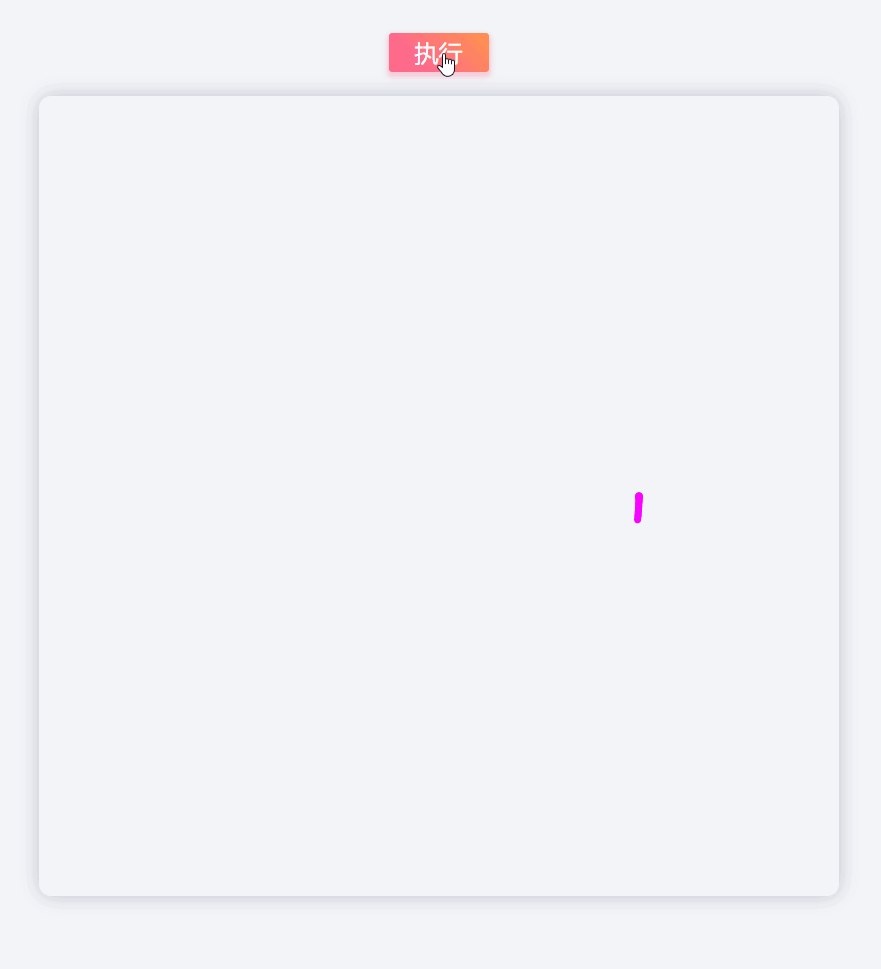`````` function handleExecute() {
// 获取canvas元素
const canvas = document.querySelector('#myCanvas')
// 获取canvas渲染上下文
const ctx = canvas.getContext('2d')

// 设置线条样式
ctx.lineWidth = 7
ctx.lineJoin = 'round'
ctx.lineCap = 'round'

// 定义圆心的坐标点
// const center = {x: ctx.canvas.width / 2, y: ctx.canvas.height / 2}
const center = {x: 400, y: 400}
// 定义圆的半径大小
// 定义起点和终点的角度
const startAngle = 0
const endAngle = 2 * Math.PI
let prevAngle = startAngle
let nextAngle
// 第一帧执行的时间
let startTime;
// 期望动画持续的时间
const duration = 900

/*
* 动画帧绘制方法.
* currentTime是requestAnimation执行回调方法step时会传入的一个执行时的时间(由performance.now()获得).
* */
const step = (currentTime) => {
// 第一帧绘制时记录下开始的时间
!startTime && (startTime = currentTime)
// 已经过去的时间(ms)
const timeElapsed = currentTime - startTime
// 动画执行的进度 {0,1}
let progress = Math.min(timeElapsed / duration, 1)
progress = Easing.Cubic.In(progress)
// 绘制方法
const draw = () => {
// 创建新的路径
ctx.beginPath()
// 计算这一帧中圆弧应该到达的角度
nextAngle = startAngle + (endAngle - startAngle) * progress
// 创建一段圆弧
ctx.arc(center.x, center.y, radius, prevAngle, nextAngle, false)
// 设置渐变的颜色
ctx.strokeStyle = `rgba(\${81 + 171 * Math.abs(1 - progress * 2)}, \${160 - 160 * Math.abs(1 - progress * 2)}, \${255},1)`
// 把这一帧的圆弧绘制出来
ctx.stroke()
//将prevAngle更新为这一帧中的nextAngle给下一帧使用
prevAngle = nextAngle
}
draw()

if (progress < 1) {
requestAnimationFrame(step)
} else {
console.log('动画执行完毕')
}
}

requestAnimationFrame(step)
}
``````

``````// 计算这一帧中圆弧应该到达的角度
nextAngle = startAngle + (endAngle - startAngle) * progress
``````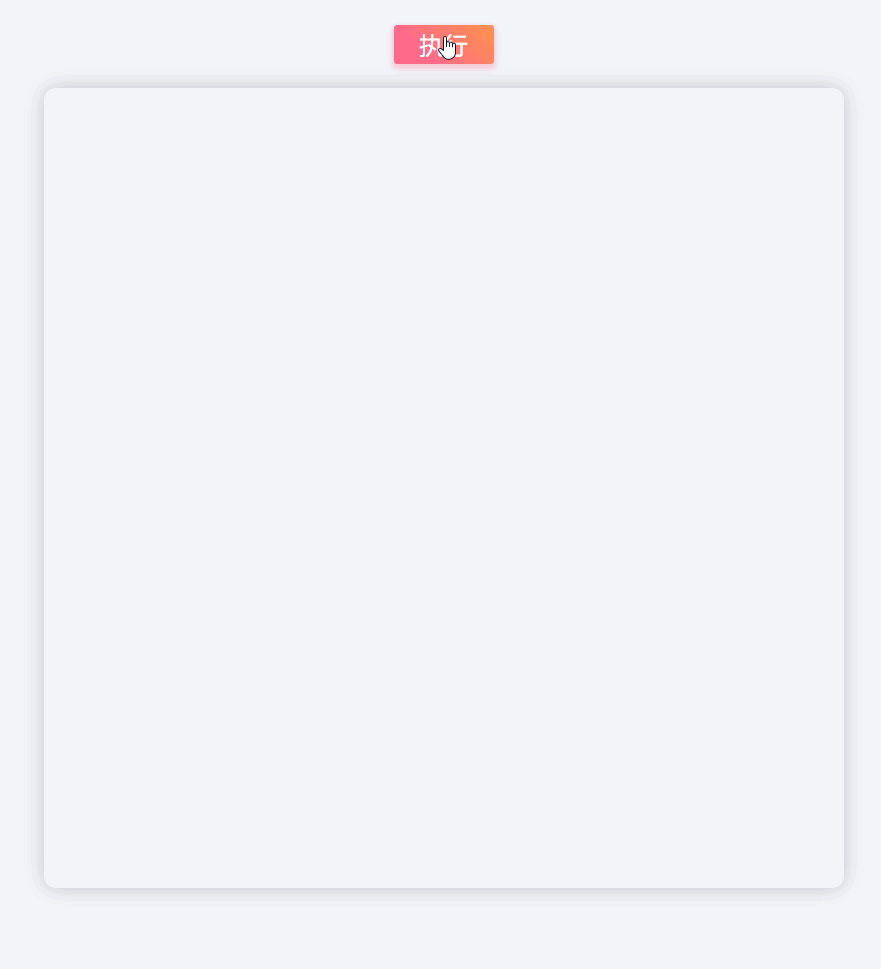gif有点掉帧了

codepen传送门codepen.io/channinghan…

## 贝塞尔曲线

canvas中有二次三次****贝塞尔曲线，本身使用它有一定的难度，但有足够的耐心你将可以用来绘制复杂而有规律的图形。

`cp1x,cp1y`为一个控制点，`x,y为`结束点

`cp1x,cp1y`为控制点一，`cp2x,cp2y`为控制点二，`x,y`为结束点。

1. 方法一：计算出贝塞尔曲线上的点，并且将足够多的点用直线连接起来。
2. 方法二：通过观察分析贝塞尔曲线的特征，将部分贝塞尔曲线中的控制点找出来，据此画出这些部分贝塞尔曲线。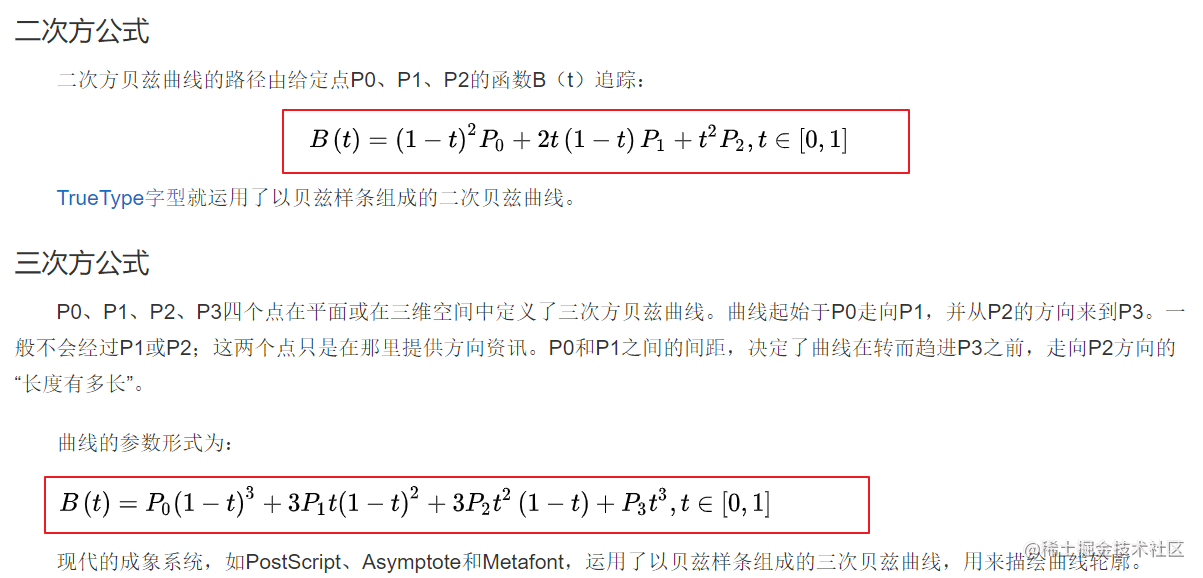P0就是起点坐标（canvas中默认为当前路径的起点），P2(二次贝塞尔)或P3（三次贝塞尔）则是终点坐标，其余中间的是控制贝塞尔曲线的控制点坐标

### 二次贝塞尔曲线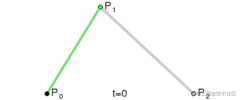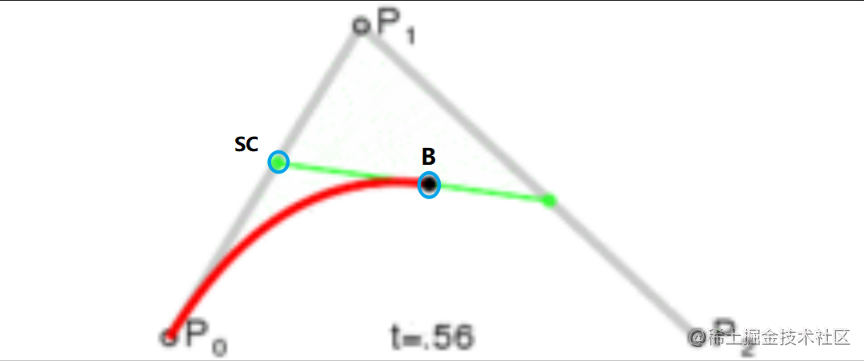``````sc.x = p0.x + p1.x - p0.x
sc.y = p0.y + p1.y - p0.y
``````

``````function handleExecute() {

// 计算出子控制点的坐标
function calSC(t) {
SC.x = p0.x + (p1.x - p0.x) * t
SC.y = p0.y + (p1.y - p0.y) * t
}

// 计算出子贝塞尔曲线的终点
function calB(t) {
B.x = Math.pow(1 - t, 2) * p0.x + 2 * t * (1 - t) * p1.x + Math.pow(t, 2) * p2.x
B.y = Math.pow(1 - t, 2) * p0.y + 2 * t * (1 - t) * p1.y + Math.pow(t, 2) * p2.y
}

// 获取canvas元素
const canvas = document.querySelector('#myCanvas')
// 获取canvas渲染上下文
const ctx = canvas.getContext('2d')

// 设置线条样式
ctx.strokeStyle = 'rgba(81, 160, 255,1)'
ctx.lineWidth = 4
ctx.lineJoin = 'round'

// 第一帧执行的时间
let startTime;
// 期望动画持续的时间
const duration = 1000

// 起点
const p0 = {x: 100, y: 500}
// 控制点
const p1 = {x: 200, y: 100}
// 终点
const p2 = {x: 700, y: 500}
// 子控制点(这里初始化的坐标不重要，先设置成p0的值)
const SC = {...p0}
// 子贝塞尔曲线上的终点(这里初始化的坐标不重要，先设置成p0的值)
let B = {...p0}

// 先画一条完整的贝塞尔曲线以验证贝塞尔曲线动画的准确性
ctx.beginPath()
ctx.moveTo(p0.x, p0.y)
ctx.strokeStyle = '#e3e3e3'
ctx.stroke()

// 随便画个眼睛（不重要）
function drawEye(color) {
ctx.beginPath()
ctx.strokeStyle = color
ctx.arc(p0.x + 100, p0.y - 50, 50, 0, 2 * Math.PI, false)
ctx.stroke()
ctx.moveTo(p0.x + 300, p0.y - 50)
ctx.arc(p0.x + 250, p0.y - 50, 50, 0, 2 * Math.PI, false)
ctx.stroke()
}

drawEye('rgb(227, 227, 227)')

/*
* 动画帧绘制方法.
* currentTime是requestAnimation执行回调方法step时会传入的一个执行时的时间(由performance.now()获得).
* */
const step = (currentTime) => {
// 第一帧绘制时记录下开始的时间
!startTime && (startTime = currentTime)
// 已经过去的时间(ms)
const timeElapsed = currentTime - startTime
// 动画执行的进度 {0,1}
let progress = Math.min(timeElapsed / duration, 1)

// 绘制方法
const draw = () => {
ctx.beginPath()
ctx.moveTo(p0.x, p0.y)
// 计算并更新B和SC的坐标
calB(progress)
calSC(progress)
// 用直线将刚刚moveTo中的点连接到(nextX,nextY)上
ctx.strokeStyle = `rgba(\${171 * (1 - progress) + 81}, \${160 * progress}, \${255},1)`
ctx.stroke()
// 眼睛渐变色
drawEye(`rgba(\${227 - (227 - 81) * progress}, \${227 - (227 - 160) * progress}, \${255},1)`)
}
draw()

if (progress < 1) {
requestAnimationFrame(step)
} else {
console.log('动画执行完毕')
}
}

setTimeout(() => {
requestAnimationFrame(step)
}, 1000)
}
``````

``````ctx.quadraticCurveTo(SC.x, SC.y, B.x, B.y)
``````

codepen传送门codepen.io/channinghan…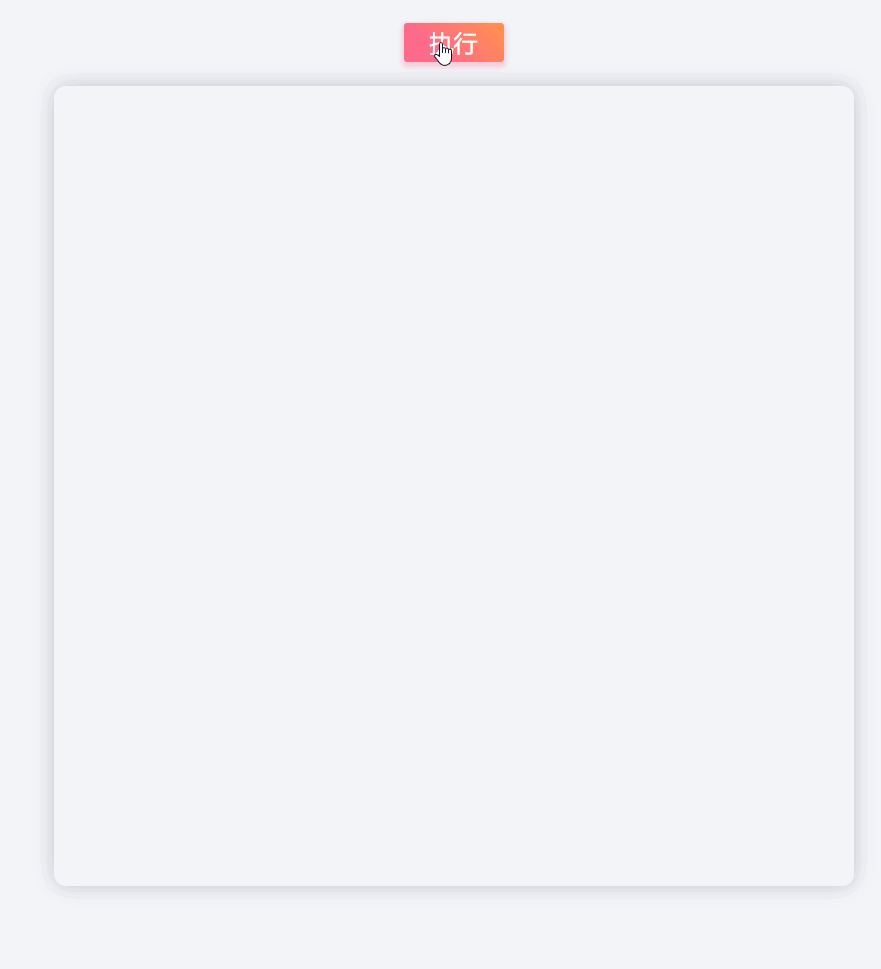god bless🙏

### 三次贝塞尔曲线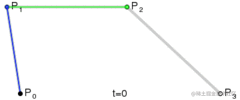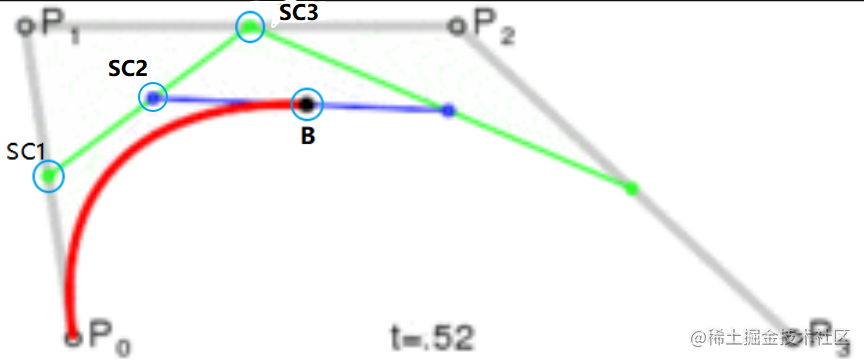``````// 计算出子控制点1的坐标
function calSC1(t) {
SC1.x = p0.x + (p1.x - p0.x) * t
SC1.y = p0.y + (p1.y - p0.y) * t
}

// 计算用于计算子控制点2的坐标的点坐标
function calSC3(t) {
SC3.x = p1.x + (p2.x - p1.x) * t
SC3.y = p1.y + (p2.y - p1.y) * t
}

// 计算出子控制点2的坐标
function calSC2(t) {
SC2.x = SC1.x + (SC3.x - SC1.x) * t
SC2.y = SC1.y + (SC3.y - SC1.y) * t
}

// 计算出子贝塞尔曲线的终点
function calB(t) {
B.x = Math.pow(1 - t, 3) * p0.x + 3 * t * Math.pow(1 - t, 2) * p1.x + 3 * p2.x * Math.pow(t, 2) * (1 - t) + Math.pow(t, 3) * p3.x
B.y = Math.pow(1 - t, 3) * p0.y + 3 * t * Math.pow(1 - t, 2) * p1.y + 3 * p2.y * Math.pow(t, 2) * (1 - t) + Math.pow(t, 3) * p3.y
}
``````

``````ctx.beginPath()
ctx.moveTo(p0.x, p0.y)
// 计算并更新B和SC1、SC2、SC3的坐标
calB(progress)
calSC1(progress)
calSC3(progress)
calSC2(progress)
// 用三次贝塞尔曲线将刚刚moveTo中的点连接到B上
ctx.bezierCurveTo(SC1.x, SC1.y, SC2.x, SC2.y, B.x, B.y)
ctx.strokeStyle = `rgba(\${171 * (1 - progress) + 81}, \${160 * progress}, \${255},1)`
ctx.stroke()
``````

codepen传送门codepen.io/channinghan…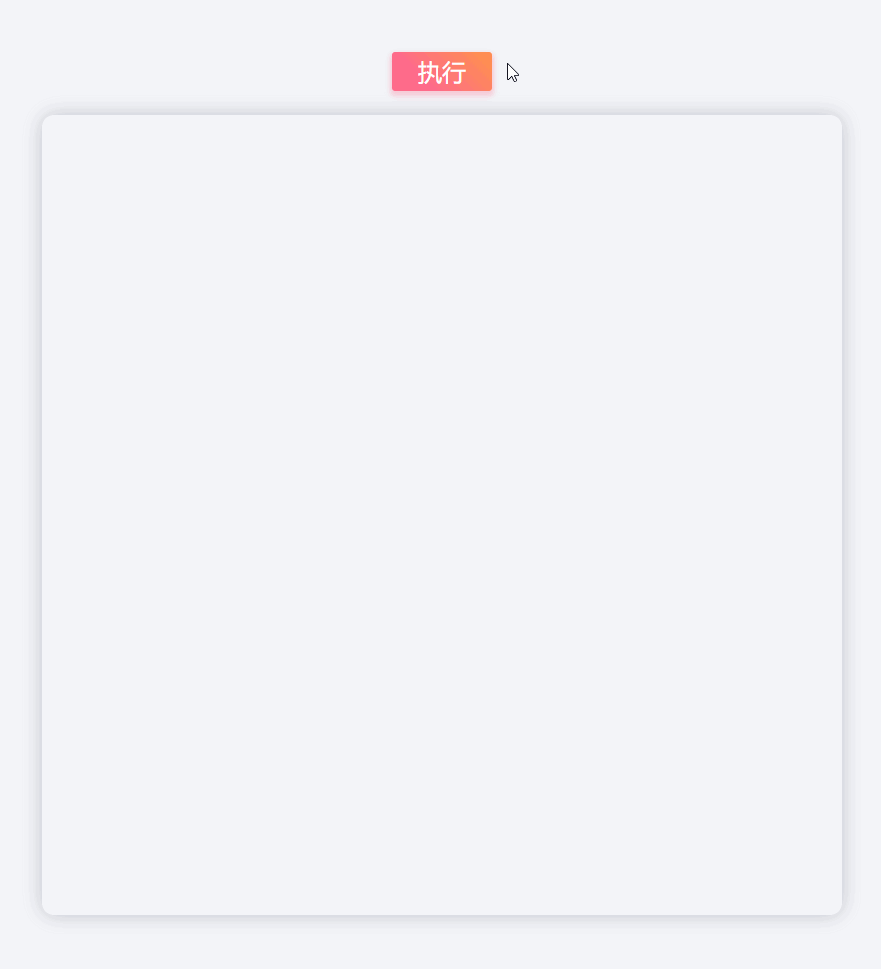god bless again🙏